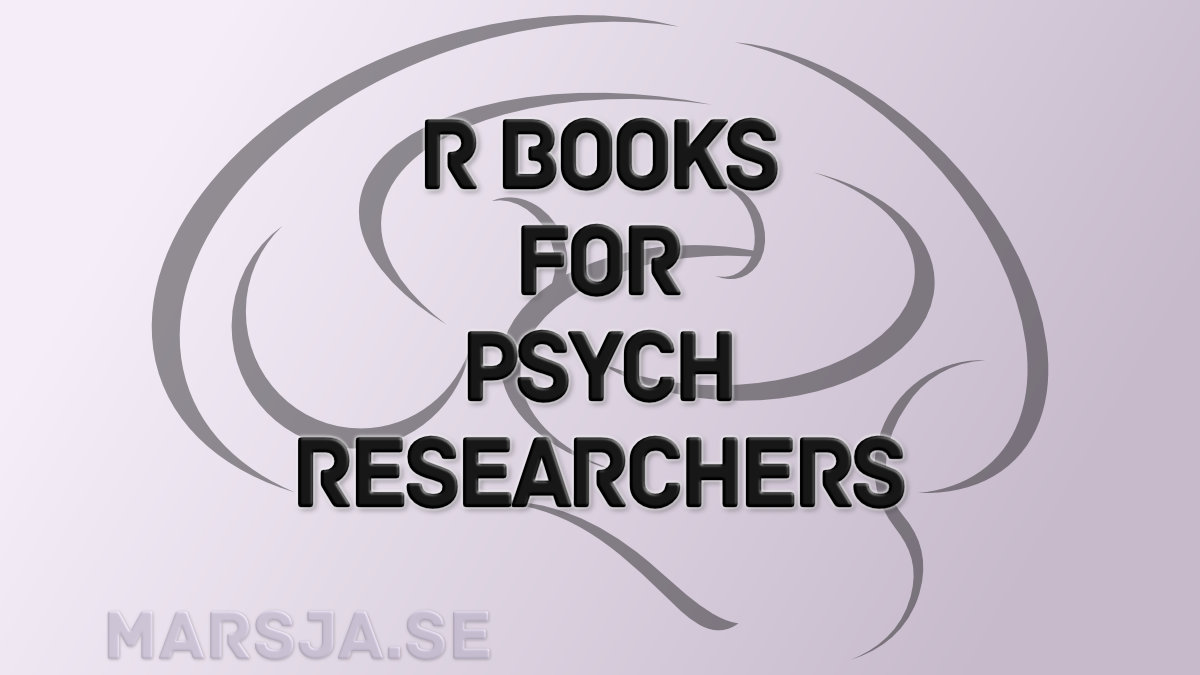1 Share

R is a free and open-source statistical programming environment. Being open-source and free it has a large and helpful online community (for instance, see StackOverflow).  When I went from carrying out analysis in SPSS to do them in R, I searched for good books targeted to Psychologists.   In this post, you will find four great books using R as a statistical tools. All four books are useful and good for Psychologists that want to learn R.
The first book, Discovering Statistics Using R, is probably a really good start if you are an undergraduate student that have no experience of either programming or statistics. If you, on the other hand, already have some experience with at least programming, the next two books cover statistics from intermediate to advanced level. Finally, the last book is, at the moment, free and is also a great introduction to statistics and R statistical environment. Most of them will cover basics such as data visualization (e.g., scatter plots), carrying out descriptive statistics, creating dummy variables in R, and more advanced data analysis methods, of course.

## Great R Books

#### Discovering Statistics Using R (Field, Miles, & Field)

Andy Fields book “Discovering Statistics Using SPSS” is incredibly popular in my department. In fact, it was the first statistics book I bought that was not a coursebook.
“Discovering Statistics Using R” uses the same pedagogical and humorous approach to the subject (i.e., learning statistics using R).
It covers basic statistical and research concepts, how to explore and visualize data, basic uni-variate (e.g., t-test, correlation, ANOVA) as well as some multivariate statistics (e.g., MANOVA, & Factor Analysis). Emphasizes the application of statistics without leaving theory out. This may not be considered an R book, as it focuses on statistics but I highly recommended this an introduction to statistics and R.

#### The Foundations of Statistics: A Simulation-based Approach (Vasisthth & Broe)

“The Foundations Of Statistics” focus is on statistics in the linguistics, psychology, and cognitive science disciplines.  This book covers basic statistical concepts (e.g., power, ANOVA, linear mixed-effects/multilevel models). “The Foundations of Statistics” has a simulation-based approach and all simulations in the book can be carried out using R. Recommended for advanced undergraduate and graduate students. Although this book, as with Discovering Statistics using R, is not merely a book about doing data analysis with R, you will also get a very good introduction to statistics but you may need some prior programming experience.

#### Advances in Social Science Research Using R (Lecture Notes in Statistics) (Edited by Vinod)

I have not read this book yet, but it seems promising:

This book covers recent advances for quantitative researchers with practical examples from social sciences. The twelve chapters written by distinguished authors cover a wide range of issues–all providing practical tools using the free R software.

Note that this book covers Social Science and, again, may not be considered an R book. However, there are some interesting statistical techniques to learn (e.g., Quantile Regression).

#### Learning Statistics with R

“Learning Statistics with R”  is a work in progress. The first draft is available for free. If you prefer to read a “real” book, there is also the option to buy a relatively cheap hard copy.
The book is written by Danielle Navarro for her introductory statistics class (for psychologists). It covers background on why we learn statistics and a short introduction of research design. The book progresses into introducing the reader to R, working with data (descriptives statistics, visualization, etc.), and statistical theory. It covers parametric tests such as z-test, t-test, ANOVA, repeated measures ANOVA, and linear regression. Oh, on the topic of linear regression “Learning Statistics with R” also covers Bayesian Statistics in one chapter.

There are probably more R books with a focus on Psychology or Social Sciences, but I am not aware of them. If you happen to know a good book, maybe covering a more advanced and/or specific topic, please leave a comment.1 Share